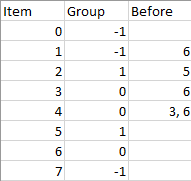## Algorithm

Problem Name: 1203. Sort Items by Groups Respecting Dependencies

There are `n` items each belonging to zero or one of `m` groups where `group[i]` is the group that the `i`-th item belongs to and it's equal to `-1` if the `i`-th item belongs to no group. The items and the groups are zero indexed. A group can have no item belonging to it.

Return a sorted list of the items such that:

• The items that belong to the same group are next to each other in the sorted list.
• There are some relations between these items where `beforeItems[i]` is a list containing all the items that should come before the `i`-th item in the sorted array (to the left of the `i`-th item).

Return any solution if there is more than one solution and return an empty list if there is no solution.

Example 1:```Input: n = 8, m = 2, group = [-1,-1,1,0,0,1,0,-1], beforeItems = [[],,,,[3,6],[],[],[]]
Output: [6,3,4,1,5,2,0,7]
```

Example 2:

```Input: n = 8, m = 2, group = [-1,-1,1,0,0,1,0,-1], beforeItems = [[],,,,,[],,[]]
Output: []
Explanation: This is the same as example 1 except that 4 needs to be before 6 in the sorted list.
```

Constraints:

• `1 <= m <= n <= 3 * 104`
• `group.length == beforeItems.length == n`
• `-1 <= group[i] <= m - 1`
• `0 <= beforeItems[i].length <= n - 1`
• `0 <= beforeItems[i][j] <= n - 1`
• `i != beforeItems[i][j]`
• `beforeItems[i] `does not contain duplicates elements.

## Code Examples

### #1 Code Example with Javascript Programming

```Code - Javascript Programming```

``````
const sortItems = function (n, m, group, beforeItems) {
const graph = Array.from({ length: m + n }, () => [])
const indegree = Array(n + m).fill(0)
for (let i = 0; i < group.length; i++) {
if (group[i] == -1) continue
graph[n + group[i]].push(i)
indegree[i]++
}
for (let i = 0; i < beforeItems.length; i++) {
for (const e of beforeItems[i]) {
const a = group[e] === -1 ? e : n + group[e]
const b = group[i] === -1 ? i : n + group[i]
if (a === b) {
// same group, ingroup order
graph[e].push(i)
indegree[i]++
} else {
// outgoup order
graph[a].push(b)
indegree[b]++
}
}
}
const res = []
for (let i = 0; i < n + m; i++) {
if (indegree[i] === 0) dfs(res, graph, indegree, n, i)
}
return res.length === n ? res : []

function dfs(ans, graph, indegree, n, cur) {
if (cur < n) ans.push(cur)
indegree[cur] = -1 // mark it visited
for (let next of graph[cur] || []) {
indegree[next]--
if (indegree[next] === 0) dfs(ans, graph, indegree, n, next)
}
}
}
``````
Copy The Code &

Input

cmd
n = 8, m = 2, group = [-1,-1,1,0,0,1,0,-1], beforeItems = [[],,,,[3,6],[],[],[]]

Output

cmd
[6,3,4,1,5,2,0,7]

### #2 Code Example with Python Programming

```Code - Python Programming```

``````
class Solution:
def sortItems(self, n: int, m: int, group: List[int], beforeItems: List[List[int]]) -> List[int]:
def topo_sort(points, pre, suc):
order = []
sources = [p for p in points if not pre[p]]
while sources:
s = sources.pop()
order.append(s)
for u in suc[s]:
pre[u].remove(s)
if not pre[u]:
sources.append(u)
return order if len(order) == len(points) else []

# find the group of each item
group2item = collections.defaultdict(set)
for i in range(n):
if group[i] == -1:
group[i] = m
m += 1
# find the relationships between the groups and each items in the same group
t_pre, t_suc = collections.defaultdict(set), collections.defaultdict(set)
g_pre, g_suc = collections.defaultdict(set), collections.defaultdict(set)
for i in range(n):
for j in beforeItems[i]:
if group[i] == group[j]:
else:
# topological sort the groups
groups_order = topo_sort([i for i in group2item], g_pre, g_suc)
# topological sort the items in each group
t_order = []
for i in groups_order:
items = group2item[i]
i_order = topo_sort(items, t_pre, t_suc)
if len(i_order) != len(items):
return []
t_order += i_order
return t_order if len(t_order) == n else []
``````
Copy The Code &

Input

cmd
n = 8, m = 2, group = [-1,-1,1,0,0,1,0,-1], beforeItems = [[],,,,[3,6],[],[],[]]

Output

cmd
[6,3,4,1,5,2,0,7]